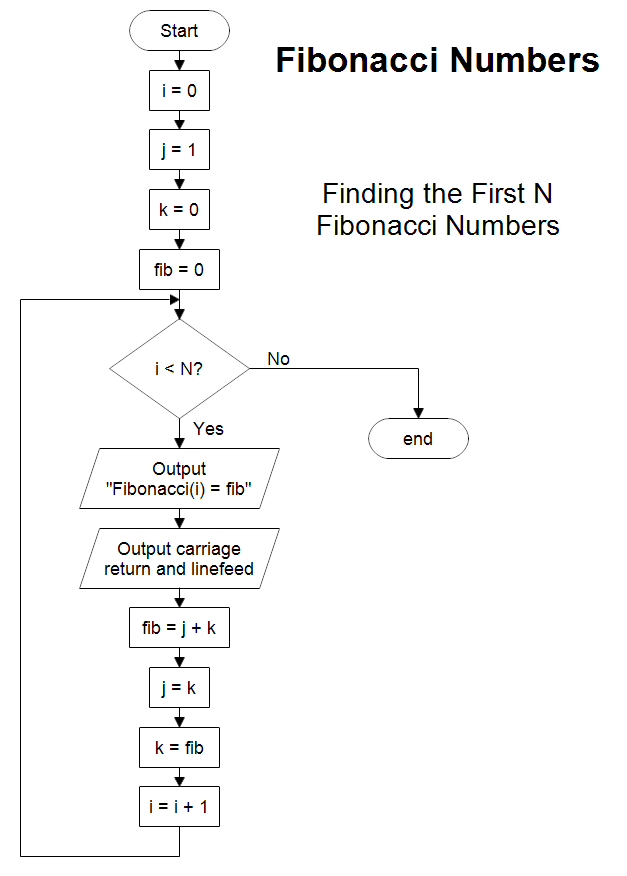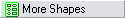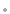Professional Flowcharting SoftwareFibonacci Numbers

Description
This flowchart answers the question "How do you draw a flowchart to calculate the first N Fibonacci numbers?" If N is 20, then you get Fibonacci numbers 0 through 19. The Fibonacci series are the numbers:

0, 1, 1, 2, 3, 5, 8, 13, 21, 34, 55, 89, 144, ...

By definition the first two numbers are:
Fibonacci(0) = 0
Fibonacci(1) = 1

The next number is always the sum of the previous two.
Fibonacci(n) = Fibonacci(n-1) + Fibonacci(n-2)

Fibonacci(2) = 0 + 1 = 1
Fibonacci(3) = 1 + 1 = 2
Fibonacci(4) = 1 + 2 = 3
Fibonacci(5) = 2 + 3 = 5
Fibonacci(6) = 3 + 5 = 8
Fibonacci(7) = 5 + 8 = 13
Fibonacci(8) = 8 + 13 = 21
Fibonacci(9) = 13 + 21 = 34
Fibonacci(10) = 21 + 34 = 55
Fibonacci(11) = 34 + 55 = 89
Fibonacci(12) = 55 + 89 = 144
Fibonacci(13) = 89 + 144 = 233
Fibonacci(14) = 144 + 233 = 377
...

The output statements in the flowchart show the value of i and the Fibonacci number fib. You would print or display one line and then go to the next as shown below.

Fibonacci(0) = 0
Fibonacci(1) = 1
Fibonacci(2) = 1
...

The flowchart above goes through each number in the series. You could use it to find an arbitrary number. However, there might be a faster way. If you just wanted the Fibonacci Number for some index i, Fibonacci(i), do you have to go through the series starting with 0, or can you think of a direct solution? Given the input number i, draw a flowchart that quickly finds the output Fibonacci(i).
Drawing Instructions
If you haven't already done so, first download the free trial version of RFFlow. It will allow you to open any chart and make modifications.

Once RFFlow is installed, you can open the above chart in RFFlow by clicking on fibonacci-numbers.flo. From there you can zoom in, edit, and print this sample chart. It is often easier to modify an existing chart than to draw it from scratch.

To draw this chart without downloading it, run RFFlow and click on the More Shapesbutton. Scroll to the Flowcharting folder and click the plus sign to open it. Click the Basic Flowcharting stencil and then click the Add Stencil button.
RFF Electronics
PO Box 1244Loveland CO 80539-1244 USAPhone 970 663 5767www.rff.comrff@rff.com